# The SAT Math II Subject Test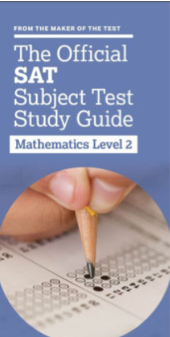The Math II Subject Test from the College Board is a 50 question test with a 60-minute time allotment. The score range is from 200 to 800 points. Each correct answer earns 1 point while ¼ of a point is subtracted for each incorrect answer. All of the questions are multiple choice with 5 options. A graphing calculator is necessary to accurately solve many of the questions. Questions about Algebra and Functions and Plane and Coordinate Geometry make up a large percentage of the test followed by Trigonometry and Data Analysis (statistics and probability). While calculus may be helpful in answering a few questions, the test does not require knowledge of calculus topics. The test is generally offered 6 times a year (January, May, June, October, November, and December). Be sure to check with the College Board as some locations do not offer the test on every date.

## Scaled Score

Your raw score is converted to a scaled score. The scale differs from test to test. In light of the scale, you do not necessarily need to answer every question correctly to score an 800. A raw score in the mid-40 range can be a reported score of 800.

## Formulas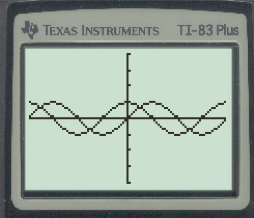A reference page is provided which lists a few formulas such as the volume of a right circular cone and the surface area of a sphere. It is vital that you know the more general formulas that would have been provided on the SAT such as the Pythagorean Theorem, relationships of sides and angles of a 30-60-90 and 45-45-90 triangle, etc.  You should know the rules for exponents and logarithms, the law of sines and cosines, and an assortment of standard trigonometry identities. Often it is helpful to have some more complicated formulas programmed in your calculator (e.g., the law of cosines).

## Practice Tests

The College Board has published four Math II Subject Tests. While some questions in the Barron’s Subject Test book are more difficult than you may find on the actual test and some, possibly, not within the scope of the actual test, their material ranks high on the list of practice questions. Other publishers (Kaplan, The Princeton Review, McGraw-Hill, Ivy Global) are worth considering as well. The key: practice, practice, practice!

Take a full-length practice test before you begin preparing. Take the test under real test conditions (sitting, timed). This test will help you understand your weakest area and where you need to focus your efforts. How much preparation time and how many practice tests you should take depends on your math and test-taking skills.## A Few Tips & Important Considerations: Math II Subject Test

1. ### Asymptotes:

Ex. 1: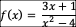Ex. 2: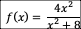Ex. 3:Finding the vertical asymptote(s) is very easy. Set the denominator equal to 0.

Therefore, in Ex. 1 above, if – 4 = 0; = 4; x= ±2. In Ex. 2 above, there are no vertical asymptotes because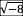is not a real number. In Ex. 3 above, the vertical is x = 1.

Finding the horizontal asymptote(s) is (almost) horrible because there are conditions that apply.

(a) If the numerator’s degree is < than that of the denominator, there is a horizontal asymptote at y=0 (i.e., the x-axis).

(b) If the numerator’s degree is > than that of the denominator, there is NO horizontal asymptote (that doesn’t rule out a slant asymptote).

(c) If the numerator and denominator have the same degree, the horizontal asymptote is ratio of the leading coefficients.

In Ex. 1 above, since the larger degree is in the denominator, the horizontal is at y = 0.

In Ex. 2 above, since the degrees are the same (2), the horizontal is y = 4/1 = 4.

In Ex. 3 above, since the degree of the numerator (2) is larger than the degree of the denominator (1), there isn’t a horizontal asymptote (but there is a slant asymptote).

1. ### Logarithms:

What is the equivalent to: Log(a²– b²) ?

Keep in mind that factoring never leaves youregardless of the math course. Consequently, this question can be rewritten as log[(a+b)(a-b)]. Since logs are exponents, they follow the exponent rules. Therefore, the product of like bases with exponents is the sum of the exponents. That means the equivalent expression is:  log(a + b) log(a – b).

1. ### Equation of a Sphere:

Given:  x²+ y²+ z²+ 2x – 4y – 10 = 0

Problems like this extend the notion of the equation of a circle to 3-dimensions. You could be asked for the coordinates of the center and/or the radius of the sphere. The solution requires grouping and completing the square.

First, move the constant to the right side: x²+ y²+ z²+ 2x – 4y = 10

Second, group the like terms:  (x²+ 2x) + (y²– 4y) + z²= 10

Third, complete the square for the x- and y-terms:  (x²+ 2x + 1) + (y²– 4y + 4) + z²= 10+1+4

*Don’t forget to add the two values to the right side after completing the square!

Now, (x+1)²+ (y-2)²+ z²= 15.

Therefore, the center is (-1, 2, 0) and the radius is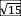1. ### Law of Sines/Cosines:

In ΔABC, if = 1, = 4, and ∠C = 30°, find the length of c.

For many, it’s helpful to quickly sketch the figure: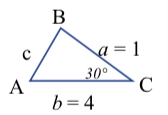Artistic skill doesn’t count, but be as accurate as you can.

Deciding between the two laws necessitates knowing that the Law of Cosines applies to problems when you have two sides and the included angle as is this case.

Therefore, c²= a²+ b²– 2ab∙cos(30°) →c²= 1+16-8∙0.866  (if you need your calculator, remember to change the MODE to degrees; otherwise, it’s helpful to remember the fundamental functions). The cos(30°) =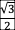.  Then, c²≈ 10.07, so the answer is ≈ 3.17. A “trap” answer option would be 10.07 if you forgot to take the square root!

1. ### Probability:

If you were to randomly form a committee of 5 people when you have a choice of 6 men and 9 women, what is the probability that the committee consists of 3 men and 2 women?

Remember that probability is: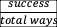. The number of total ways is the combination of selecting 5 people out of 15.  That is 15C5=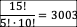. To be successful, you would have to select 3 men from 6 and 2 women from 9:  6C3  and 9C2.  The probabilities in an and condition are multiplied together (in an or condition they are added together).

6C3 and 9C2  = 20∙36 = 720.  Then, 720/3003 = 240/1001 [combinations and permutations can also be found on your TI calculator under MATH →PRB.

This site uses Akismet to reduce spam. Learn how your comment data is processed.